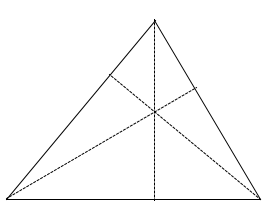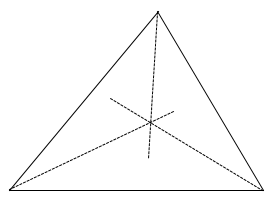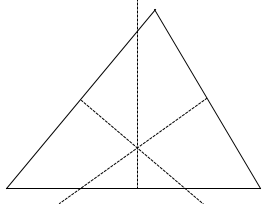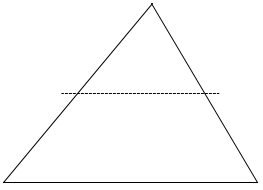# Lines and Segments of Triangles

Description for calculating lines and segments of triangles

## Lines and Segments

There are three lines and segments associated with triangles, with a point of intersection.

## Altitudes

• An altitude of a triangle is a perpendicular segment from a vertex to its opposite side.

• An altitude can be drawn from any vertex, therefore, every triangle has three altitudes.

• When the three altitudes of a triangle intersect, their point of intersection is called an orthocenter.

• An orthocenter can occur inside, on, or outside the triangle, depending on the types of angles.## Medians

• The median of a triangle is a line segment joining a vertex to the midpoint of its opposite side.

• Because a median can be drawn from any vertex, every triangle has three medians.

• Unlike altitudes, medians don’t form a right angle with the side they intersect.

• The medians divides the triangle into two smaller triangles of equal area.

• The point of concurrency of the three medians of a triangle is called the centroid.

• The centroid is always inside the triangle and is two thirds of the distance from each vertex to the midpoint of the opposite side.Ps the centroid, it dividing the bisectors in 2: 1 ratio

The lengths of the bisectors of the triangle $$a$$, $$b$$ and $$c$$ are calculated using the following formulas

$$\displaystyle Pa=\frac{\sqrt{2(b^2+c^2)·a^2}}{2}$$
$$\displaystyle Pb=\frac{\sqrt{2(c^2+a^2)·b^2}}{2}$$
$$\displaystyle Pc=\frac{\sqrt{2(a^2+b^2)·c^2}}{2}$$

## Angle Bisectors

• An angle bisector in a triangle is a segment that is drawn from a vertex and cuts the vertex angle in half.

• Just like altitudes and medians, every triangle has three angle bisectors because they originate from each vertex.

• The point of concurrency of the three angle bisectors of a triangle is called the incenter.

• The incenter is always inside the triangle and is equidistant from each of the three sides of a triangle.## Perpendicular Bisectors

• A perpendicular bisector of a triangle is a line that passes through the midpoint of a side and is perpendicular to that given side.

• Every triangle has three perpendicular bisectors.

• The point of concurrency of the three perpendicular bisectors of a triangle is called the circumcenter.

• The circumcenter can be inside, on, or outside the triangle and is equidistant from the vertices of a triangle.## Midsegments

• A midsegment of a triangle connects the midpoints of two sides of a triangle

• The Triangle Midsegment Theorem states that a midsegment of a triangle is parallel to one side of the triangle, and its length is half the length of that side